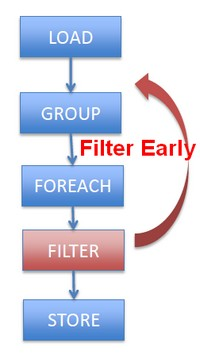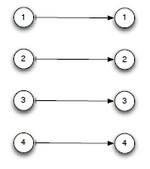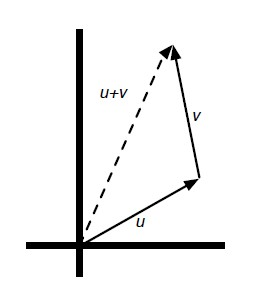# (Function|Operator) - Algebraic (Laws|properties) - Axioms

Discover More(Function | Operator | Map | Mapping | Transformation | Method | Rule | Task | Subroutine)

Section computable function. A function is a callable unit that may be called: a procedure, a subrontine a routine, a method (belong to an objectmacrocomputablalgorithreusable blocargumentdevelopment...(Function|Operator) - Commutative property (Order doesn’t matter)

Commutative is an algebraic law. By commutative property , order in the operand doesn’t matter. Addition a + b = b + a Multiplication x . y = y . x commutative(Function|Operator) - Symmetry

A function is symmetric if: SeAlgebraic Optimization

Same as: Algebraic Laws: (+) identity: x+0 = x (/) identity: x/1 = x () distributes: (nx+ny) = n(x+y) () commutes: xy = yx Apply rules 1,3,4,2 and we get Two operations instead of five,...Function - Identity

An identity function is an algebraic law that defines when the output of the function is equal to the input. identity function. For any domain D: each domain element d map to itself: Addition...Linear Algebra - Vector Space (set of vector)

A vector space is a subset of the set of function representing a geometric objectpassing through the origin. A vector space over a field F is any set V of vector : with the addition and scalar-multiplication...Linear Algebra - Vector Vector Operations

Vector Vector Operations: (also known as addition or substraction) (Scaling) Using operator overloading, you can compute this operations with the following syntax. Operation Syntax...Number - Gf(2) - Galois Field 2

Galois Field 2 has just two elements: 0 and 1 Usual algebraic laws still hold, e.g. multiplication distributes over addition Addition is like exclusive-or: + 0 1 0 0 1 1 1 0 vector addition...